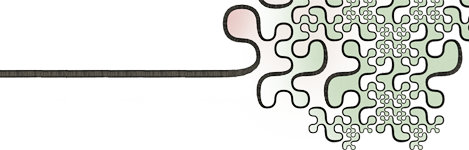at the Internet Archive

Two hundred pages of color images
in 8.5"x8.5" paperback print
A Taxonomy of Fractology
A chapter from Brainfilling Curves by Jeffrey Ventrella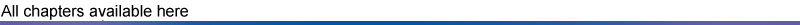1 Horror Vacui     2 A Very Patient Turtle Who Draws Lines     3 A Taxonomy of Fractology     4 Gallery of Specimens
 Root 2 Family Root 3 Family Root 4 Square Grid Family Root 4 Triangle Grid Family
 Root 5 Family No Root 6! Root 7 Family Root 8 Family
 Root 9 Square Grid Family Root 9 Triangle Grid Family Root 10 Family Root 12 Family
 Root 13 Square Grid Family Root 13 Triangle Grid Family Root 16 Square Grid Family Root 16 Triangle Grid Family
 Root 17 and Beyond... 5 My Brain Fillith Over 6 References 7 AcknowledgementsThere are many (many) kinds of fractals. The most popular fractals are the images generated with math equations iterated in the complex plane, such as the Mandelbrot Set and the Julia Sets, and all their amazing variations and magnifications.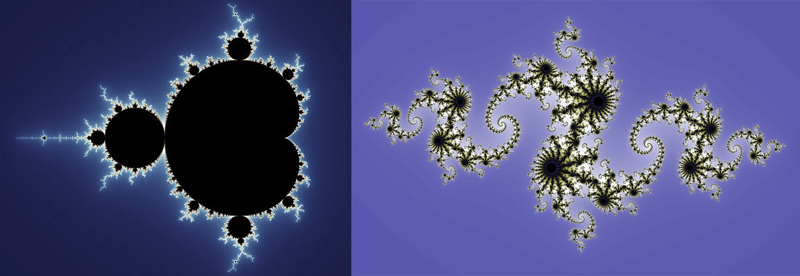These fractals are generated by calculating a mathematical function for every pixel location of a 2D grid, using the x and y coordinates of the grid as input values, to determine a color value. Mandelbrot and Julia sets use complex number equations. They have been studied extensively, and reproduced with endless variety on the internet. Fractals of this type are beautiful, complex, and amazing. But they do not easily lend themselves to learning the basic geometry of fractals, as a form of visual construction. This is why I am so enamored with self-similar fractal curves - as tools to think with; as visual objects to apply various geometrical ideas and processes from other domains. The process by which they are constructed can be explained to non-mathematicians. And if you are curious enough, and especially if you are willing to do a little bit of programming, it can lead to discovery upon discovery. The fractal generation technique that I use in this book is a variation of "Koch construction". It is based on the idea of taking a figure consisting of connected segments, and recursively replacing each segment with a copy of the whole figure. This is how the Koch curve is generated, and it is also how an infinite number of other possible fractal curves can be generated. Now, before I go on, I want to describe a few of the methods that have things in common with Koch construction. In some cases, these might be considered variations, or different approaches, to Koch construction. They are: * Node-replacement curves * Iterated function systems (IFS) * Branching Fractal Trees * L-Systems

 Node-Replacement Curves One of the most well-studied techniques for generating plane-filling curves is a process that is similar to Koch construction, except that it is based on node replacement instead of edge replacement. This technique has been explored by mathematicians and hobbyists for centuries. Peano, Hilbert, Moore, and Wunderlich, are among the mathematicians who have described such curves. Below is an example of using this technique to generate a well-known curve with a generator of four nodes: the Hilbert Curve . It is based on the fact that a square can be tiled with four smaller squares. Take a look at how this simple generator is copied four times, transformed, and connected to form the shape at right: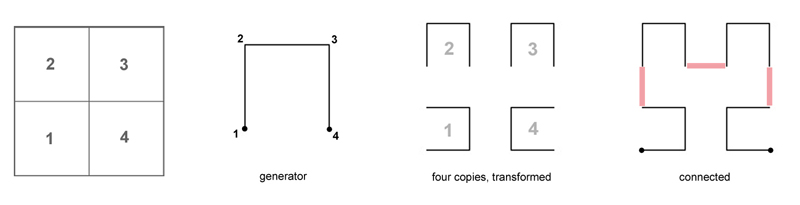Now let's see six progressive fractalizations (with node connections added). The Hilbert curve is shown at left. On the right is a variation called the Moore curve.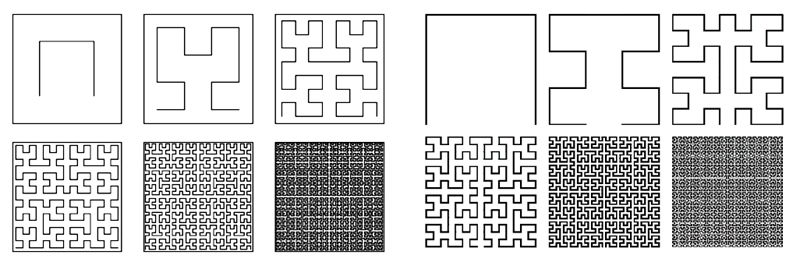Node-replacement curves are generated by replacing tiles with smaller copies of the generator, while edge-replacement curves are generated by replacing the segments of a generator with smaller copies of the generator. One important consequence of node-replacement is that it requires connective segments to be added at each stage, to keep the curve unbroken, as we just saw above. Some examples of similar curves are shown below: a curve attributed to Walter Wunderlich (a); a curve attributed to Peano (b); and the Z-Order (Lebesgue) Curve (c). The Sierpinski Curve (d) is shown here because it is similar, however it is of a different class: it uses a different kind of connectivity at each stage.Gary Teachout  came up with several fractal curves using a similar technique. A few are shown here.

 By the way, the resulting shape doesn't have to be a simple polygon; it can have a fractal boundary. Here's a generalized technique for generating curves with node-replacement: start with a collection of tiling polygons, like squares, and connect each of the tile centers with line segments, as shown below at left. Now...fractalize! Notice in this example that although there are five tiles in the initial figure, there are only four line segments. This fact is why connecting lines are required at each iteration (As we saw in the Hilbert curve, four square tiles are connected using three line segments).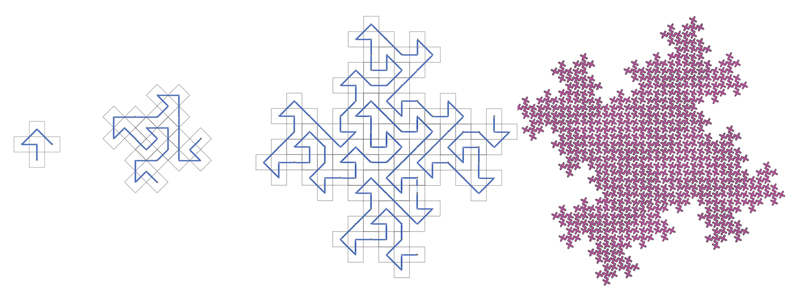The above curves fractalizes to the same shape as a well-known fractal curve introduced by Mandelbrot (at right). I'll be describing this curve later on.

 Iterated Function Systems A more general class of fractal techniques is a process known as "iterated function systems" (IFS), conceived by John Hutchinson , and developed further by Michael Barnsley , and others. An intuitive way to describe IFS is to start with an initial image or piece of geometry, and to replace it with 2 or more copies of itself. Each copy has some transformation applied to it (e.g., rotation, translation, scaling). With IFS, copies of the original shape are progressively reduced in size repeatedly until they are essentially "atomized" as points, whose union creates the resulting shape. As long as the copies are scaled to be smaller than the original geometry, the ultimate result is always a "dust" - the number of visual elements goes to infinity, and their sizes become infinitely small, thus, the original geometry could be anything, basically. Take the example below of the symbol for Pi, a hammer, and a poodle. This trio of elements is copied twice, and each copy is scaled to 70% its original size. Using the bottom of the hammer as the pivot point, one copy is rotated -40 degrees, and the other is rotated 60 degrees, and also translated down and to the right a bit. All these translations happen in the frame of reference of the transformed copy. This example shows 8 iterations of this process, and you can see that a fractal has emerged with its own peculiar shape - a shape that may not have been predicted from applying the transformation only once.Here is another picture that illustrates this process. In this picture, the original geometry consists of two gray squares, shown at left with a larger outlined square for reference. Each gray square is replaced with the original geometry, using the same relation that the gray squares have with the larger outlined square.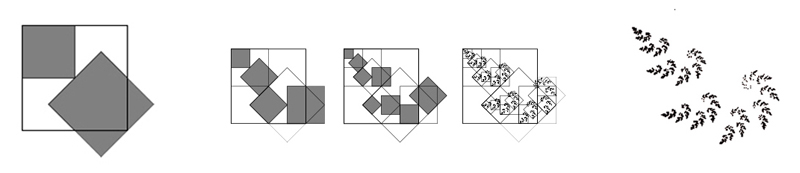The fern is a classic natural form that is often used to demonstrate IFS.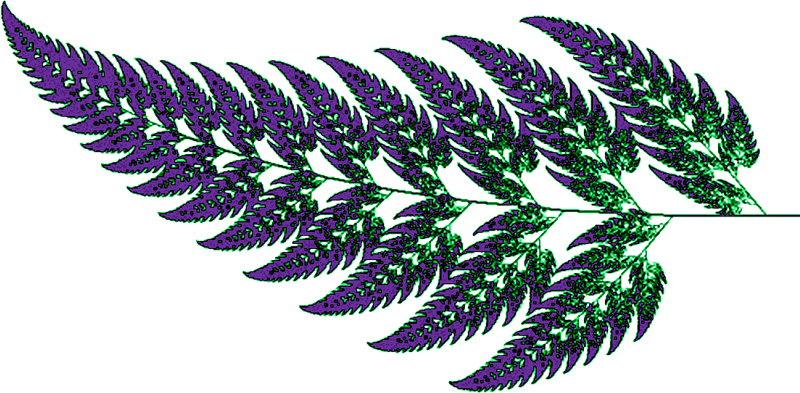IFS can be seen as a more general way to perform Koch construction. Just consider the segments of a Koch generator as the original elements. Instead of poodles and hammers, you start with straight lines, which happen to transform at each stage so that they always form a connected chain.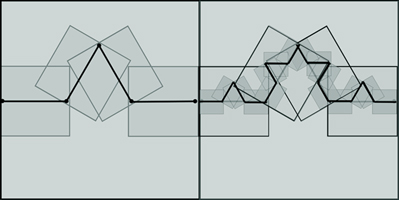Branching Fractal Trees When visualizing data structures with many branchings, such as classifications of biological species, one ends up with a fractal-like shape, especially if there is deep hierarchy. This is indicated below in a Tree of Life drawn in 1866 by the German biologist and illustrator Ernst Haeckel (a).Branching fractals differ from Koch fractal curves in two primary ways: (1) they contain branch-points (obviously), and (2) they include the whole hierarchy of ancestry used to calculate each level: trunk, branch, stem, and all. Koch construction, on the other hand, replaces each parent generator with offspring copies when a new teragon is calculated, leaving only the smallest, most detailed segments. The classic fractal tree (b) uses a single angle at each branch point, with a uniform scaling < 1.0 of offspring segment lengths. When the angle is widened, we get a shape like (c). If the angle is 90 degrees (and if the length scaling is 1/(root2)) it becomes the H-tree (d) - a plane-filling, self-avoiding fractal. Irregular branching forms can be created in a variety of ways, including diffusion-limited aggregation (e): start with a seed crystal particle surrounded by free-floating particles moving randomly. If a floating particle comes in contact with the seed, it sticks, thus extending the seed crystal, which gradually grows into a fuzzy branching fractal form.

 L-Systems In 1968, a botanist by the name of Astrid Lindenmayer devised a way of describing the growth of plants. This has come to be known as "L-systems". They are recursive "string-rewriting systems", used to model branching forms, embryological development, and many self-similar fractal geometries. Consider the following L-system description used to draw the Koch curve: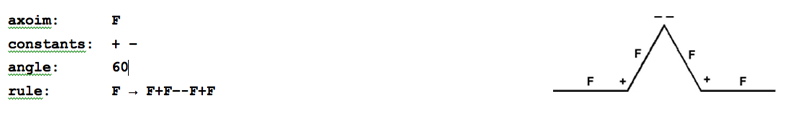The axiom "F" means "draw a line segment". The symbol "+" means turn left 60 degrees. And the symbol "-" means turn right 60 degrees. We start with the axiom "F", and we apply the rule, which replaces "F" with "F+F--F+F". This results in a teragon 1 Koch curve. Notice the use of "--" to represent turning right twice, which makes a 120 degree right turn. Now we apply the rule again, replacing all instances of "F" with "F+F--F+F". This gives us:If we apply the rule a third time, replacing all instances of "F" with "F+F--F+F", we get:Once this string has been grown, it can be given to the turtle as a single list of instructions, and the turtle goes to work. This is only the very beginning of what L-systems can do! L-systems are more than just a way to make fractals; they can be used for describing (and constructing) a huge number of forms, including Hilbert curves and its variants, branching forms, and many other shapes. L-systems can even be used for modeling embryological development over time. This flexibility is because any kind of alphabet can be used (not just F's and +'s and -'s) which can stand for any transformation or operation you can imagine. L-systems provide textual representations of fractals (linear strings of alphabetical symbols that are read from start to finish). The turtle must read the entire string - like a novel or a score for a piano sonata - and follow it from start to finish. Although L-systems are elegant, terse, and very expressive, I prefer to put the turtle into the middle of the algorithm, so to speak, and engage the fractal growth process in a concrete, procedural way - to conceptually enter into the geometrical process of fractalization - as a visual-spatial activity. My turtle is not reading a score; it is performing a fractal dance - holding in its memory all the recursive levels and transformations required to do the dance. Now that I have described a few alternate techniques and variations used to make fractals, I want to now focus on the particular technique that I have devised. It is based on Koch construction, as described by Mandelbrot, but it has some new and unique differences, which I think might make it easy to understand. Most importantly, my scheme allows all Koch-constructed fractal curves to be placed into a taxonomy, allowing us to see relations and family types, and to explore variations. Now, the first thing I have to explain is the backdrop upon which all these wonderful specimens are generated: the grid.

 Two Grids All plane-filling fractal curves in my scheme fit into one of two kinds of grids: triangle or square. The distance between grid points is exactly 1, in either case. The most common fractal curve angles and lengths in these specimens are shown at right, associated with each grid.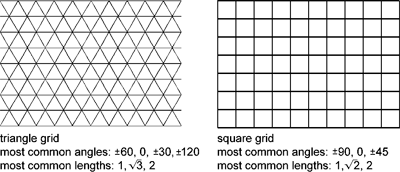Consider two curves: the Koch curve and a similar one with a square bump (sometimes called the "Square Koch"). Not only do the generators fit snuggly within their grids, but all of their fractalized teragons fit snuggly as well (that is, they fit snuggly into grids with smaller and smaller cells as fractals levels increases).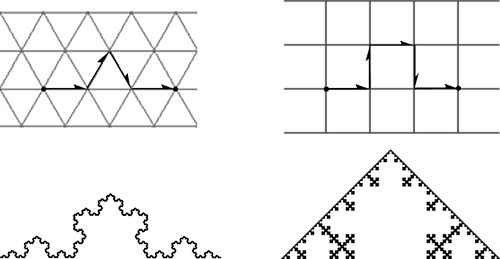Fractal Dimension Notice also that each of these two fractal generators covers a span of three grid units, from left to right. This span is called the "interval length" of the fractal generator. The Koch curve has four segments, but its squarish friend has five: it fills a bit more space - it's slightly denser. More technically, it has a higher fractal dimension. Fractal dimension extends the idea of Euclidean dimension (integer numbers) to include fractional numbers, and lots of fuzzy structures besides.Here's how we calculate the fractal dimension of a curve: we take the number of segments in the generator (call it N), and then we take the interval length of the generator (call it L). Then we calculate the fractal dimension using this equation: log N / log L In the case of the Koch curve, N=4, and L=3, and so the fractal dimension comes out to approximately 1.2618. For the square Koch, N=5, and L=3, and it's fractal dimension is approximately 1.4649. If the dimension of a fractal curve is 2 - and if it is well-behaved - it is a plane-filling curve. Families of fractal curves are determined according to how their generators fit within their grids, and what kinds of grids they occupy. Not all fractal generators are as simple as Koch and its square friend. And not all fractal generators rest horizontally on the grid. I'll give you two examples.
 Let's draw a square grid, and then refer to a grid point in the lower left corner as the origin. This will be the starting point for all fractal generators. Since all plane-filling fractal generators fit into a grid, we can be sure that the end of the generator will always fall on a grid point. Below is a square grid with two of the grid points indicated. The distance from the origin to these grid points are root2 and root5, respectively. (We know this because of the Pythagorean theorem: a2 + b2 = c2 - see diagram at right). Well, it turns out that there are two very special fractal generators that fit snuggly within these diagonal spaces. They are used to make the classic dragon curve, and the "5-dragon" (that's the name I use for a fractal I discovered many years ago).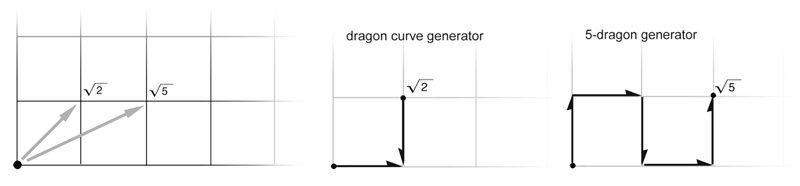Let's fractalize these two generators and see what happens: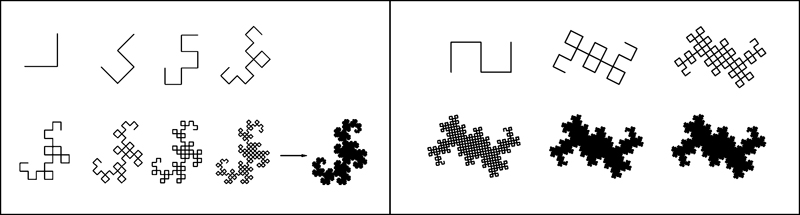What have we here? We have two plane-filling fractal curves! That means their fractal dimensions are exactly 2. And it should come as no surprise that the number of segments in each generator is exactly the square of the interval length. In other words: N = L2 Now we have a consistent scheme for finding plane-filling fractal curves. The four fractals that I have just showed you are listed in the table below, along with their associated grid type and interval length (which I call "family type"). This table represents four different family types.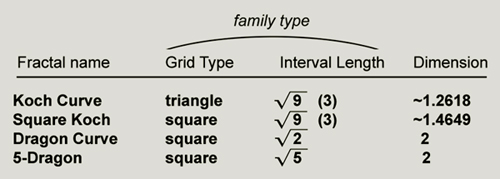Grid Distances It is easy to use the Pythagorean theorem to figure out the grid point distances from the origin in the square grid, but the triangle grid is a bit more tricky. That is because the right triangle used to calculate a2 + b2 = c2 has lengths that are not integers.
 The image at right shows how the Pythagorean theorem is used on a triangle grid to find the length of hypotenuse c, which represents the interval length of a fractal generator. Remember that all fractal generator intervals fall between two grid points. In the triangular grid, the length of horizontal leg a will always be a multiple of 0.5, and the length of vertical leg b will always be a multiple of root3/2. It turns out that with these length multiples, the value of c is always the square root of an integer. This is very convenient for my fractal family taxonomy scheme!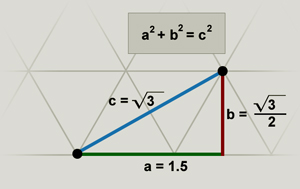Now, look at these two grids: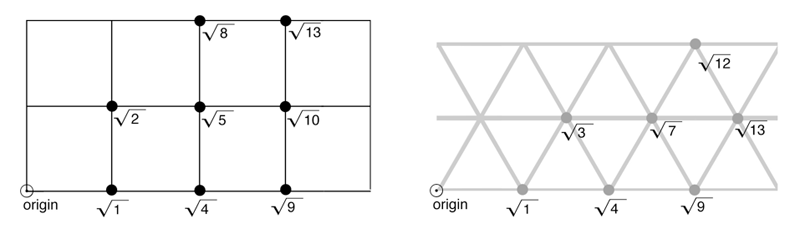The distances from the origin to some of the grid points are shown. Notice that the distances shown at the bottom row are expressed as square roots, but this is just another way of saying 1, 2, 3. The square and triangle grids provide the backdrop for identifying all the families of plane-filling fractal curves. Let's superimpose the triangle grid onto the square grid. In doing so, we see that there are many square root distances from the origin that fall on grid points (but some are missing...like 6! - and the reason is very interesting - as I will explain later).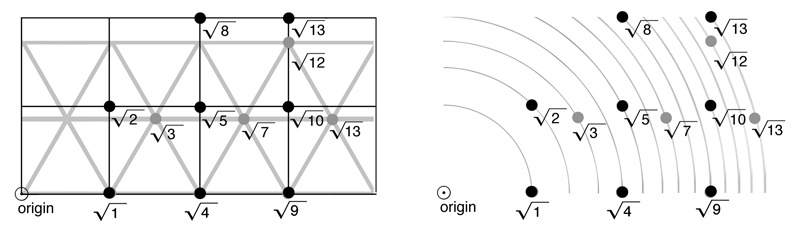You may have noticed that I am only showing the distances within a certain clustered area in the grid. The reason is because there is no need to consider any grid points that lie outside a certain pie slice, as shown below. For instance, in the square grid at right, an octant is highlighted, with several distances shown. All these distances could be found at grid points in the other seven octants, so we only need one octant to identify all the families. Similarly, in the triangular grid I have highlighted a pie slice occupying one twelfth the space. Any triangle-grid family type can be represented in this slice.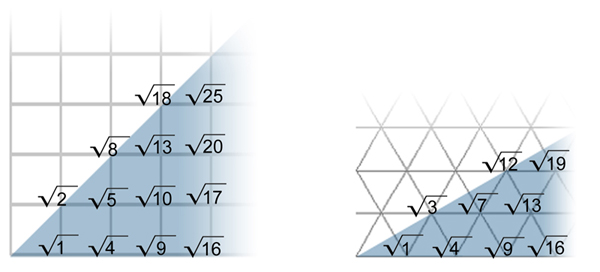Ventrella Notation This grid-view of fractal generators comprises the basis for how I categorize all plane-filling fractal curves. In The Fractal Geometry of Nature, Mandelbrot specifies all fractal generators as existing within a horizontal interval of one unit. The illustration below uses the Gosper curve as an example of how my notation scheme is slightly different than the one Mandelbrot used. Instead of specifying the generator within the unit interval such that its endpoints lie on a horizontal line, I place it within a grid, and orient it so that its second endpoint lies on the grid location associated with its family type. This causes its interval length L to traverse a portion of the grid. My notation makes it visually more apparent that the Gosper curve is a member of the root7 family type.I should point out one thing about Mandelbrot's choice to place all generators on a horizontal interval of unit 1. This normalizes the generator so that it is easier to comprehend (and compute) the mathematical transformation of that generator into smaller copies that are then placed onto itself. The unit vector represents a normalized generator segment. My scheme may be less elegant in terms of mathematical expression, but it affords a way to classify generators within a large taxonomy, whereby the placement of the generator within the grid is the basis of the classification. Interestingly, my software algorithms necessarily transform all generators to a representation within the unit interval - for ease of computation. So perhaps Mandelbrot representation could be seen as a necessary step, both algorithmically and conceptually. In one sense, my scheme for finding plane-filling fractal curves is simple, using the grid as a guide. However, it is not as simple as you might think (if you are novice in the fine art of fractalizing), because there are many misbehaved, self-crossing, and otherwise clumpy, hole-ridden fractals out there, obscuring the beautiful gems. And the difference between the well-behaved plane-fillers and the misbehaved ones is not something you can easily determine just by looking at a generator. For many generators, especially the ones with large interval lengths, you just have to fractalize them and find out. And you have to be patient.

 Self-avoiders and Gridfillers There are a few more points I want to make before we take the tour of the specimens. I mentioned that some fractal curves are "well-behaved", but most are not. I need to be a little more specific than that. There are four distinct ways that a fractal curve can behave, in terms of how it interacts with itself: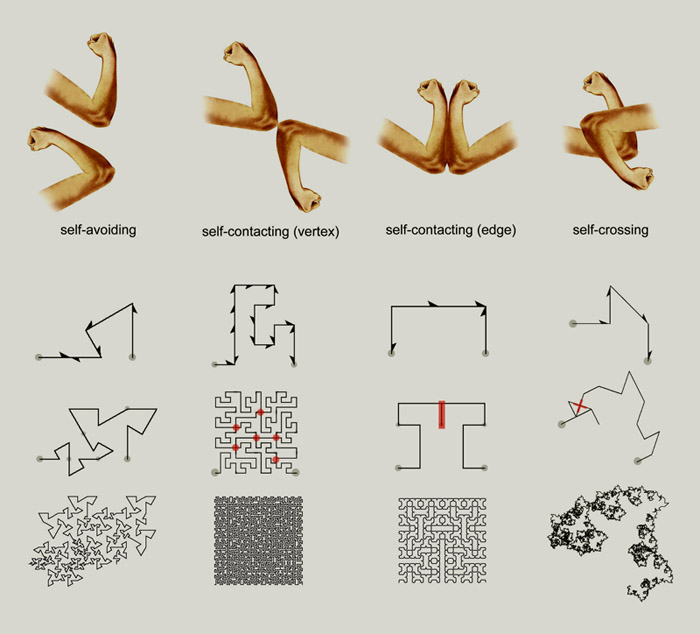Because of the recursive nature of fractalization, any self-touching or self-crossing that occurs is sure to propagate with each fractalization...approaching infinity at higher levels. But self-touching and self-crossing fractal curves are not necessarily bad. In fact, some of the most interesting-looking fractal curves cross-over themselves excessively. And if you are a fractal explorer, I encourage you to fish for whatever strikes your fancy. But there is an intellectual - and in a certain way, aesthetic - satisfaction in finding fractal curves that are well-behaved. Also, finding them is a challenge - and we do so love a challenge! So, the majority of my specimens are either self-avoiding or self-touching on their vertices. The most exciting discoveries are the "FASS curves". "FASS" stands for space-Filling, self-Avoiding, Simple, and self-Similar. I have already defined "Space-filling" and "self-avoiding". "Simple" means the curve does not cross itself, and "self-similar" means that the curve appears the same at different magnifications and rotations. The recursive operations used in Koch Construction, L-systems, and IFS insure self-similarity. Now, there is a certain class of vertex self-touching I want to mention, in which the fractal curve touches itself at every grid point within the area that it covers (except for the boundary). These are what I call the gridfillers. The dragon curve and the 5-dragon that I showed you earlier are both gridfillers. They fill a portion of the square grid. They can be described as "chunks of lattice" (in Mandelbrot's words). There are also many fractal curves that fill triangular grids, such as the Ter-dragon (shown below, with its first eight teragons). It will be described in more detail later on.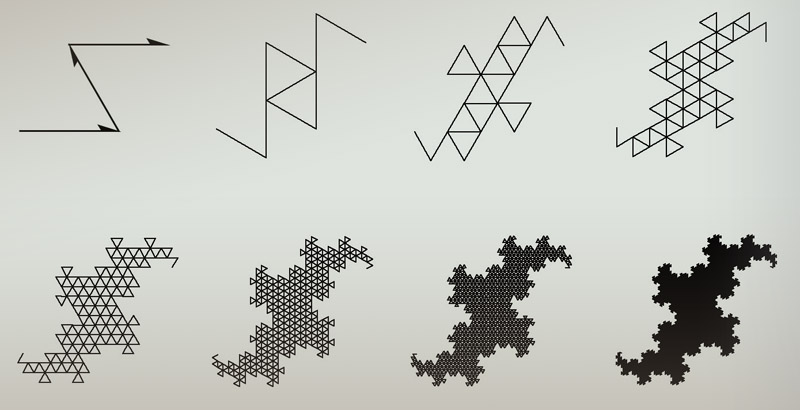Rounded Corners Since the Ter-dragon is a "chunk of lattice", it is hard to see how the curve sweeps through its body. To reveal the sweep of the curve, we can use rounded corners (more specifically: beveled, or chopped-off, corners). At the right is the Ter-dragon's 6th teragon, rendered with rounded corners. For a triangle gridfiller, chopping off the corners makes them hexagon-like rather than triangle-like. The 9-segment generator shown below lives in the square grid; chopping off its corners makes them octagon-like rather than square-like.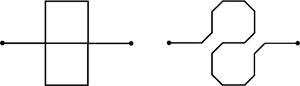You will notice that many of the specimens in this book have curly lines, and that the curls appear to be self-avoiding. That is because I have rendered them with rounded corners. So, don't get confused if you think you are looking at a specimen that is a self-avoider! To make sure it is clear, the diagrams always specify if a teragon is drawn with rounded corners.

 Splines Some of the high-resolution renderings of curves in this book employ splines, which are even smoother than the chopped-off corners used in the diagrams. The picture at right shows an example of a fractal curve whose sharp corners normally touch each other. Splines help to separate these touch points, and they also give the curve a smooth organic contour.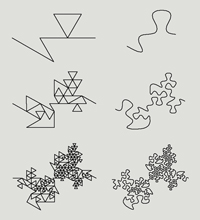How Long is a Fractal Curve? Good question! Here's the answer: Infinite. And here's why: whenever you fractalize a teragon, you multiply its overall length by some constant value which is greater than 1. You may have heard Mandelbrot's famous question, "How long is the coast of Britain?" Well, it depends on whether you are measuring it with the flight of a jet plane, the mileage recorded from a car ride, or the meandering of a crab crawling along the shore. Since a true Platonic fractal has an infinite number of fractal levels of self-similarity, the length essentially becomes infinitely long (even though it still occupies a finite area) This is illustrated below with the Dragon of Eve. This fractal curve's length is multiplied by ~1.707 at each level.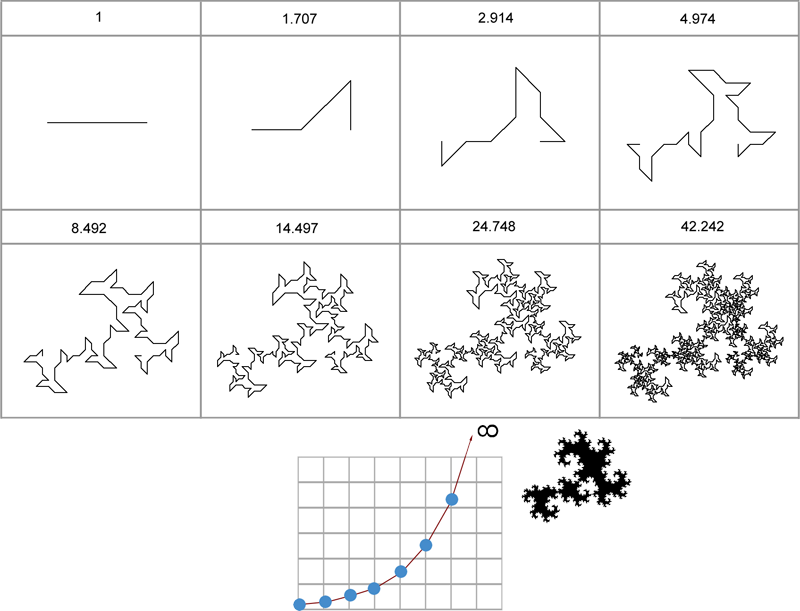Per-Tiling, Rep-Tiling, and Penrose Tiling Since a fractal curve is made of smaller copies of itself, it logically follows that a plane-filling fractal curve is a filled-in shape that is made of smaller filled-in shapes - identical to itself. This means that plane-filling fractal curves are tiling. Not only are they tiling, but they are recursively tiling. Mandelbrot called this "pertiling" (using the prefix "per" as in perfume: to thoroughly fill with fumes). These tiles are also examples of "rep-tiles". A rep-tile is a plane figure that tiles the plane and can be divided into several smaller copies of itself. Some examples are shown below at left. At right are pertilings (fractal rep-tiles) of a specimen I will show you later.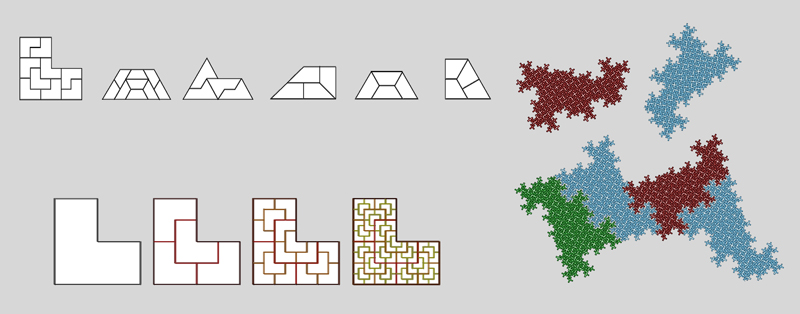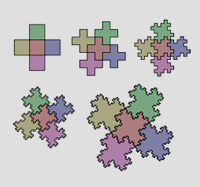Some fractal explorers, such as Tom Karzes , have designed pertilings that are a-periodic, called "Penrose Fractals". This means these tilings can extend out forever and never repeat the same pattern. One of Tom's designs is shown at right. Penrose Fractals are further described by Bandt and Gummelt , and Gelbrich .When I put two related fractal specimens together like puzzle pieces, I like to use the term pertiling - especially if they have wild fractal boundaries. Sometimes I refer to this as "mating". Why? Because only specimens of similar species can fit together. You'll see as we meet the different families of plane-filling curves that each family has its own particular type of morphology. Below are two related specimens mating. The ends of their curves touch at the bottom of the image.

 Okay, I believe we have covered enough of the basics now. In the pages that follow, I will be showing you more than 200 specimens of plane-filling curves. I have decided to leave some of the remaining interesting concepts for later, as they come up in relation to the various specimens. There's a lot more to look at and a lot more to think about. Let the fractalizing begin!1 Horror Vacui     2 A Very Patient Turtle Who Draws Lines     3 A Taxonomy of Fractology     4 Gallery of Specimens
 Root 2 Family Root 3 Family Root 4 Square Grid Family Root 4 Triangle Grid Family
 Root 5 Family No Root 6! Root 7 Family Root 8 Family
 Root 9 Square Grid Family Root 9 Triangle Grid Family Root 10 Family Root 12 Family
 Root 13 Square Grid Family Root 13 Triangle Grid Family Root 16 Square Grid Family Root 16 Triangle Grid Family
 Root 17 and Beyond... 5 My Brain Fillith Over 6 References 7 Acknowledgements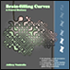Brain-filling Curves - A Fractal Bestiary by Jeffrey Ventrella Distributed by Lulu.com Cover Design by Jeffrey Ventrella Book web site: BrainFillingCurves.com ISBN 978-0-9830546-2-7 Copyright © 2012 by Jeffrey Ventrellaeyebrainbooks.comFractalCurves.com